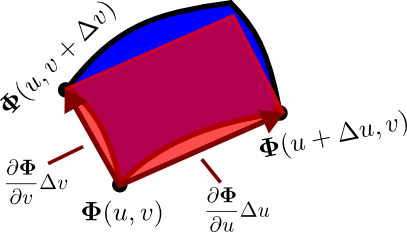# Math Insight

### Image: Parametrized surface mapping as parallelogramThe function $\dlsp$ maps a small rectangle of size $\Delta\spfv\times\Delta\spsv$ onto a “curvy rectangle” floating in three-dimensional space. We approximate the “curvy rectangle” as a parallelogram.

Image file: parametrized_surface_parallelogram.png

Source image file: parametrized_surface_parallelogram.svg
Source image type: Inkscape SVG